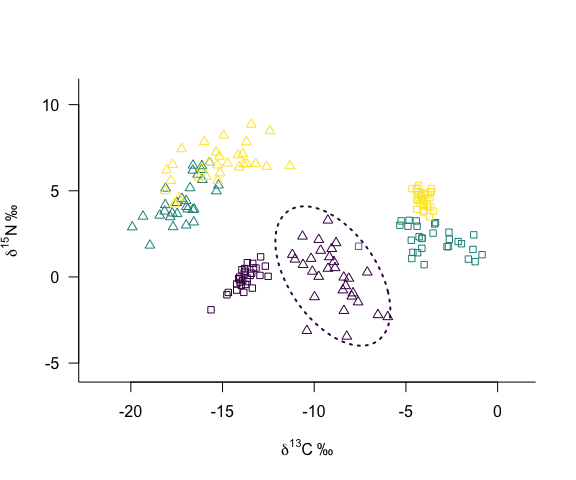# Customising Plots Manually

## Creating a Blank Plot

In the vignette Introduction to Siber we used the bundled functions plotSiberObject() to create plots with ellipses and hulls and saw how we can use plotGroupEllipses() to add some customised ellipses. Here we will look in more detail at how we can add customised elements to a blank plot by calling the underlying functions directly. Again we will use the bundled example dataset.

# remove previously loaded items from the current environment and remove previous graphics.
rm(list=ls())
graphics.off()

# Here, I set the seed each time so that the results are comparable.
# This is useful as it means that anyone that runs your code, *should*
# get the same results as you, although random number generators change
# from time to time.
set.seed(1)

library(SIBER)

# Load the viridis package and create a new palette with 3 colours, one for
# each of the 3 groups we have in this dataset.
library(viridis)
palette(viridis(3))

# load in the included demonstration dataset
data("demo.siber.data")

#
# create the siber object
siber.example <- createSiberObject(demo.siber.data)

You don’t even have to use plotSiberObject() to plot your raw data. You could plot it all yourself directly from your raw data using any points, colours and axes styles that you want. In this example, I override the default points order using the option argument points.order. See the help file for the base graphics points() function which lists the mapping of the integers 1:25 to the corresponding point type. In this case I use the order c(24, 22) which corresponds to open triangles and open squares.

Next we want to add a single ellipse, fully customised to one of our clusters of data; i.e. one of the groups within a community. Here I define the group and community using two created variables so as you could adapt this run for any group/community combination you wanted, or perhaps more suitably, write a loop to traverse all your data.

plotSiberObject(siber.example,
hulls = FALSE,
ellipses = FALSE,
group.hulls = FALSE,
bty = "L",
iso.order = c(1,2),
xlab = expression({delta}^13*C~'\u2030'),
ylab = expression({delta}^15*N~'\u2030'),
points.order = c(24,22)
)
# Call addEllipse directly on each group to customise the plot fully

# change c.id and g.id to select the group of data you want
# you could embed this in a loop easily enough if you wanted to
# set up the order of lines and simply loop through them.
c.id <- 1 # specify the community ID
g.id <- 1 # specify the group ID within the community

# NB i am using the group identifier g.id to select the colour
# of the ellipse line so that it matches the one created by
# plotSiberObject(), but you could override this if you wish.
# The function addEllipse returns the coordinates it used for plotting,
# but more than likely you dont need this information. Here I store these in
# a new variable coords for clarity, but you could just as easily call this tmp.
# See help file for addEllipse for more details on the options, but in short:
# the first two entries look up the means and covariance matrix of the data you
# specified using the group and commmunity indices above.
# m = NULL is used as we are not plotting an ellipse around the mean.
# n = 100 just determines how many points are used to draw a smooth ellipse.
# p.interval = 0.95 for a 95% ellipse of the data
# ci.mean = FALSE as we are not plotting an ellipse around the mean.
# col = your choice of colour.
# lty = your choice of line type.
# lwd = your choice of line width.
coords <- addEllipse(siber.example$ML.mu[[c.id]][ , , g.id], siber.example$ML.cov[[c.id]][ , , g.id],
m = NULL,
n = 100,
p.interval = 0.95,
ci.mean = FALSE,
col = g.id,
lty = 3,
lwd = 2)As this is just a simple base R graphics window, you can add lines, points and text as you like. You can also call the functions plotGroupHulls(siber.example) and plotCommunityHulls(siber.example) directly and customise their inputs as per the vignette Introduction to Siber.